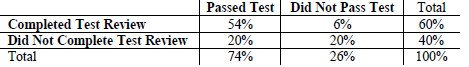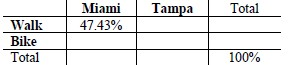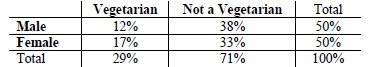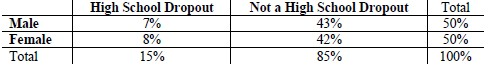# MA.912.DP.4.5Export Print
Given a two-way table containing data from a population, interpret the joint and marginal relative frequencies as empirical probabilities and the conditional relative frequencies as empirical conditional probabilities. Use those probabilities to determine whether characteristics in the population are approximately independent.

### Examples

Example: A company has a commercial for their new grill. A population of people are surveyed to determine whether or not they have seen the commercial and whether or not they have purchased the product. Using this data, calculate the empirical conditional probabilities that a person who has seen the commercial did or did not purchase the grill.

### Clarifications

Clarification 1: Instruction includes the connection between mathematical probability and applied statistics.
General Information
Subject Area: Mathematics (B.E.S.T.)
Strand: Data Analysis and Probability
Status: State Board Approved

## Benchmark Instructional Guide

### Terms from the K-12 Glossary

• Conditional relative Frequency
• Joint frequency
• Joint relative frequency
• Population

### Vertical Alignment

Previous Benchmarks

Next Benchmarks

### Purpose and Instructional Strategies

In Algebra I, students studied bivariate categorical data, displayed the data in tables showing joint and marginal frequencies, and discussed possible associations. This was extended to conditional relative frequencies in Algebra I Honors. In Math for College Liberal Arts, students calculate empirical probabilities and conditional empirical probabilities and use these calculations to determine and discuss independence.
• Instruction focuses on real-world data and interpreting the joint and marginal relative frequencies as empirical probabilities, or experimental probabilities, meaning they are based on existing, collected data. Additionally, the conditional relative frequencies will be interpreted in context as empirical conditional probabilities.
• Instruction includes discussing the differences between joint, marginal and conditional relative frequencies.
• Marginal relative frequencies Guide students to understand that the total column and total row are in the “margins” of the table, thus they are referred to as the marginal relative frequencies.
• Joint relative frequencies Guide students to connect that the word joint refers to the coming together of more than one, therefore the term joint relative frequency refers to combination of two categories or conditions happening together.
• Conditional relative frequencies Guide students to connect that the word conditional refers to imposing a constraint on one of the two variables, therefore the term conditional relative frequency refers to the percentage in a category for one variable when one has put a constraint on the other variable.
• Depending on a student’s pathway, they may not have had experience with relative frequency tables. Instruction includes making the connection between frequency and relative tables to find empirical probabilities and empirical conditional probabilities.
• For example, the relative frequency table below describes whether a student completed a test review sheet and whether they passed the test.• To determine the marginal relative frequency of those who completed the test review, one would look to the percentage of those who completed the test review, or 60%. One could say that the empirical probability of a student completing the test review is 60%.
• To determine the joint relative frequency of those who passed the test and completed the test review, one would look to the intersection of those categories within the table, or 54%. One could say that the empirical probability that a student passed the test and completed the test review is 54%.
• To determine the conditional relative frequency of those who passed the test given that they completed the review, one could take the ratio between the joint relative frequency for passing the test in the first row and the marginal relative frequency in the first row, which is 54% 60% which is equivalent to 90%. One could say that the empirical conditional probability that a student passed the test given that they completed the review is 90%.
• Instruction includes the connection between mathematical (or theoretical) probability and applied statistics. While mathematical probability deals with predicting the likelihood of an event yet to happen, applied statistics analyzes the frequency of a past event in order to make conclusions about a population. Essentially, we use probabilities to draw conclusions from given data.
• One conclusion to be analyzed from these probabilities is whether characteristics in the population are approximately independent, as seen in MA.912.DP.4.4 and MA.912.DP.4.6.
• Students may use their prior knowledge of the word independent to mean not relying on another, and believe that conditional probabilities should be different when events are independent. Emphasize that having equivalent conditional probabilities means the probability of an event is the same no matter whether the other event occurs.

### Common Misconceptions or Errors

• Students may not be able to distinguish the differences between marginal, joint and conditional relative frequencies.
• Students may not be able to distinguish the differences between frequencies and relative frequencies.

• The United States Census Bureau collected data between 2008 and 2012 on the number of workers in various cities who commuted to work by bicycle and those who walked. In looking at data for both Tampa, Florida, and Miami, Florida, there are 14,380 workers who either walk or use a bicycle to commute to work. About 47.43% of this total are people who walk to work in Miami, with about 56.04% of the people being workers in Miami. About 33.66% of those working in Tampa commute to work using a bicycle.
• Part A. Construct a relative frequency table with the given information.• Part B. Determine and interpret at least two empirical probabilities from the table in Part A. Compare your probabilities with a partner.
• Part C. Determine and interpret at least two empirical conditional probabilities from the table in Part A. Compare your probabilities with a partner.
• Part D. Are the events commuting to work via bicycle and working in Tampa independent? Explain.

### Instructional Items

Instructional Item 1
• The table displays the results of a survey of eating preferences of a sample of high school students. Use the data in the two-way frequency table below to answer the following questions.• Part A. In the context of the data, interpret the empirical conditional probability of 17/50 .
• Part B. In the context of the data, interpret the empirical conditional probability of 17/29 .

Instructional Item 2
• The table below represents dropout rates in 1970 for children between 16 and 24 years old. Are sex and dropping out of high school approximately independent?*The strategies, tasks and items included in the B1G-M are examples and should not be considered comprehensive.

## Related Courses

This benchmark is part of these courses.
1210300: Probability and Statistics Honors (Specifically in versions: 2014 - 2015, 2015 - 2019, 2019 - 2022, 2022 and beyond (current))
7912070: Access Mathematics for Liberal Arts (Specifically in versions: 2014 - 2015, 2015 - 2018, 2018 - 2019, 2019 - 2022, 2022 - 2023, 2023 and beyond (current))
1210305: Mathematics for College Statistics (Specifically in versions: 2022 and beyond (current))
1207350: Mathematics for College Liberal Arts (Specifically in versions: 2022 and beyond (current))

## Related Access Points

Alternate version of this benchmark for students with significant cognitive disabilities.

## Related Resources

Vetted resources educators can use to teach the concepts and skills in this benchmark.

## Student Resources

Vetted resources students can use to learn the concepts and skills in this benchmark.

## Parent Resources

Vetted resources caregivers can use to help students learn the concepts and skills in this benchmark.